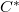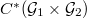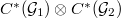﻿

### A Conditional Expectation on the Tensor Product of Exel-Laca algebras

#### Abstract

We show that the ultragraph$C^*$-algebra$C^*(\mathcal{G}_1\times\mathcal{G}_2)$ can be embedded in$C^*(\mathcal{G}_1)\otimes C^*(\mathcal{G}_2)$ as a$*$-subalgebra. We then use this fact to investigate the existence of a conditional expectation on the tensor product of Exel-Laca algebras onto a certain subalgebra.

DOI Code: 10.1285/i15900932v39n1p25

Keywords: ultragraph $C^*$-algebra; Exel-Laca algebras; conditional expectation

Full Text: PDF